## 【C++】 使用红黑树模拟实现STL中的map与set

article2023/10/3 19:28:18/

### 文章目录

• 前言
• 1. 对之前实现的红黑树进行一些补充和完善
• 1.1 析构
• 1.2 查找
• 2. STL源码中map和set的实现
• 3. 改造红黑树+封装map和set
• 3.1 红黑树结构修改
• 3.2 map、set的结构定义
• 3.3 insert的封装
• 3.4 insert测试
• 3.5 发现问题并解决
• 3.6 红黑树迭代器实现
• 3.7 封装set和map的迭代器并测试
• 3.8 map的[]重载
• 3.9 元素可以修改的问题解决
• 4. 代码展示
• 4.1 RBTree.h
• 4.2 Map.h
• 4.3 Set.h
• 4.4 Test.cpp

# 1. 对之前实现的红黑树进行一些补充和完善

## 1.1 析构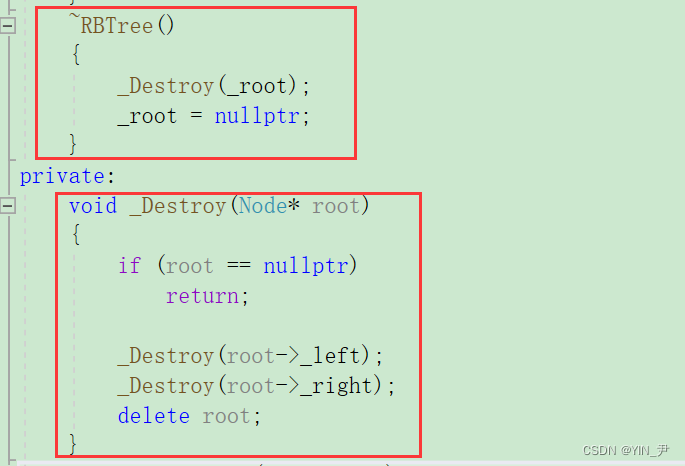## 1.2 查找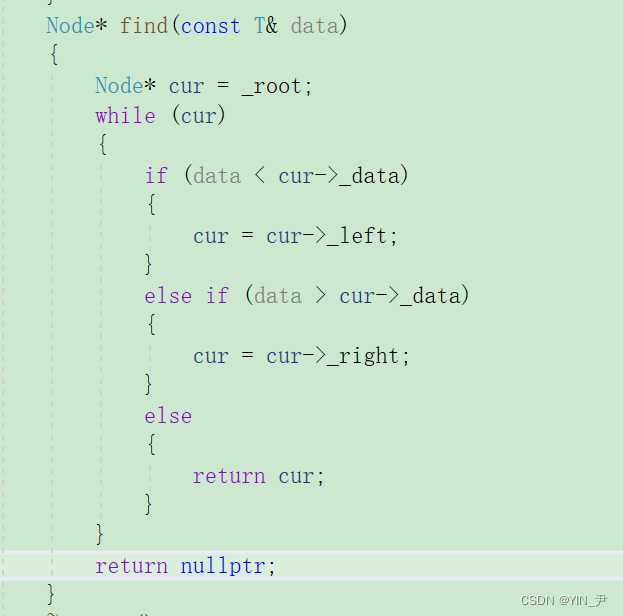# 2. STL源码中map和set的实现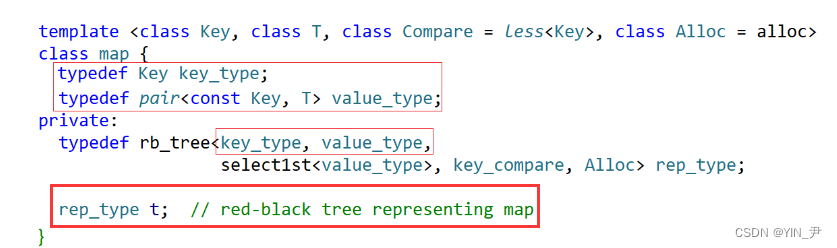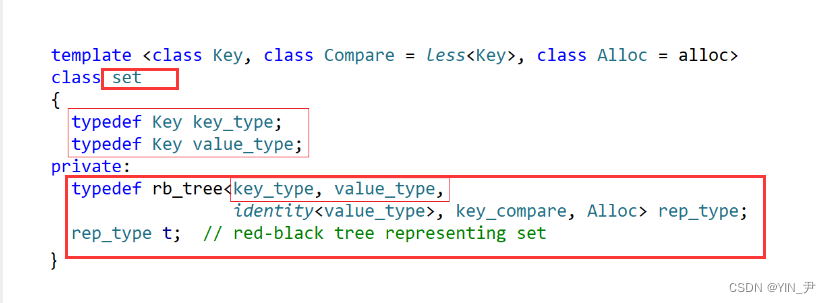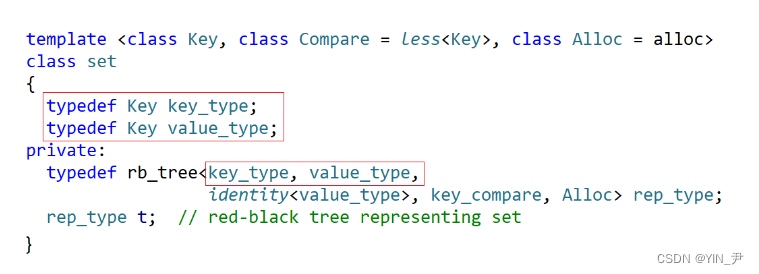set的里面虽然存的是单个值，可它除了有`key_type`之外，也有`value_type`，但是set里面的key_type和value_type都是Key的typedef，所以相当于传来两个key。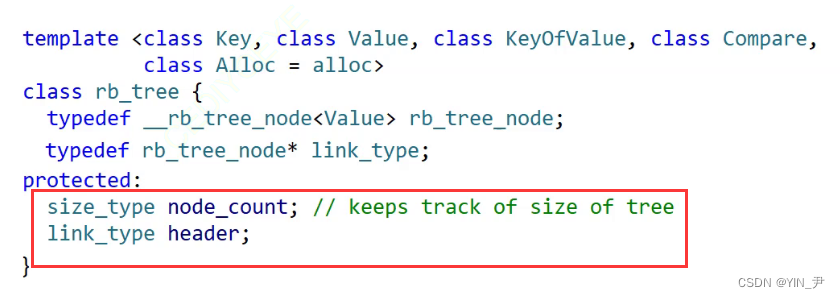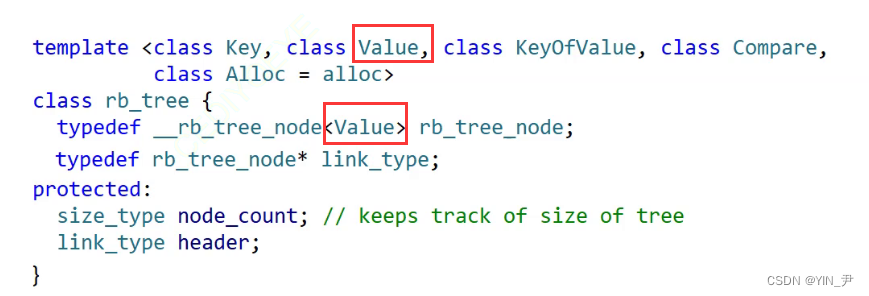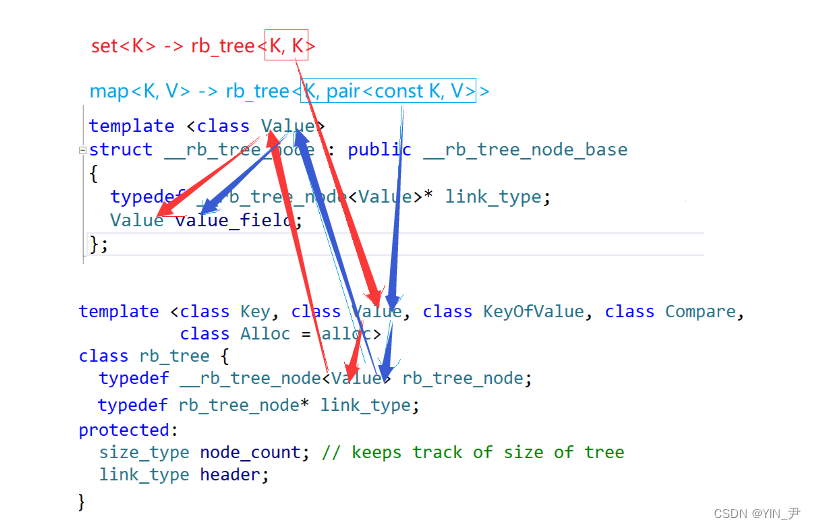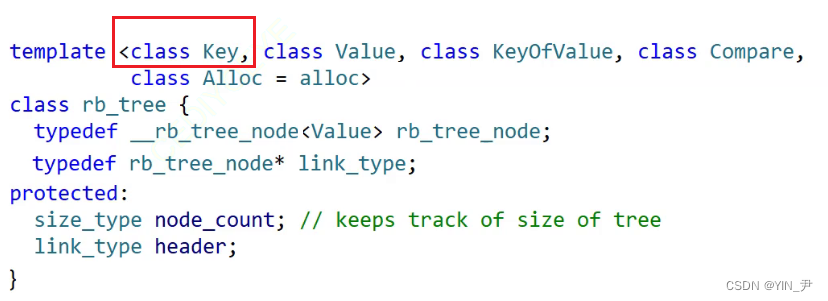🆗，对于set来说，它的查找是不是就是按结点里面存的那个key去查找的啊，返回的是对应元素的迭代器。

，因为key是唯一的，而value其实是可以重复的。

# 3. 改造红黑树+封装map和set

## 3.1 红黑树结构修改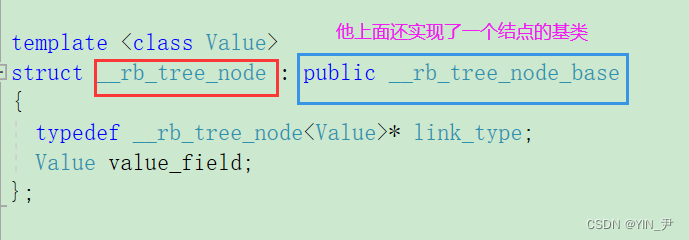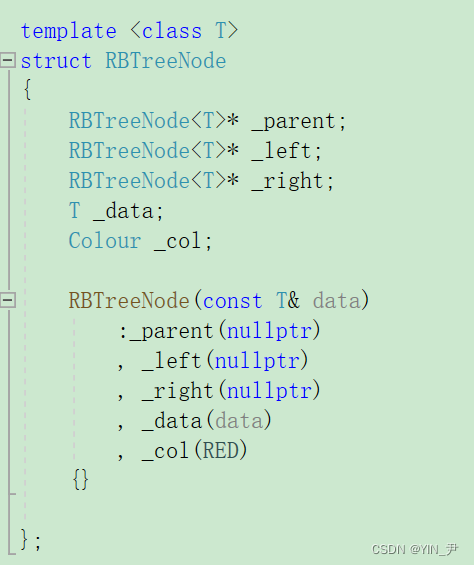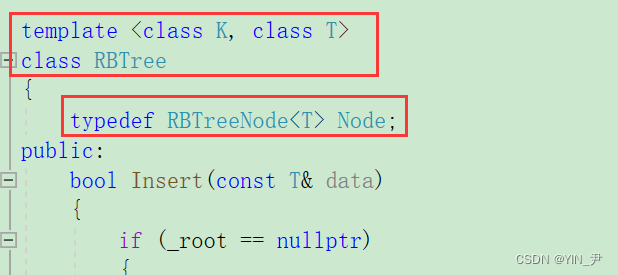## 3.2 map、set的结构定义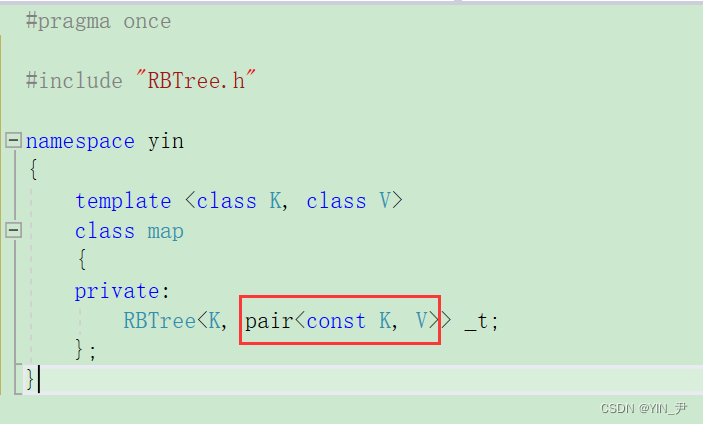## 3.3 insert的封装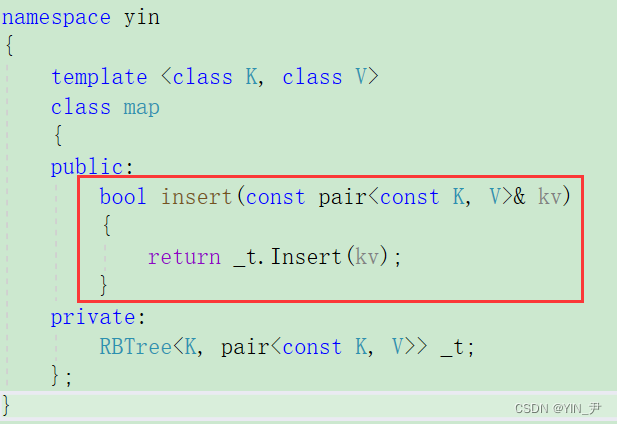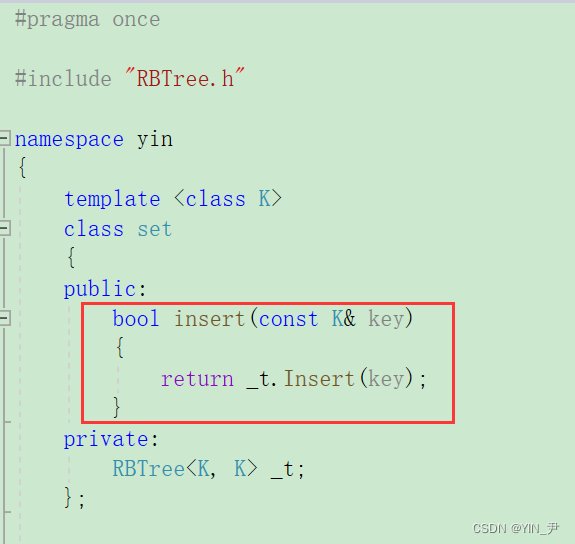## 3.4 insert测试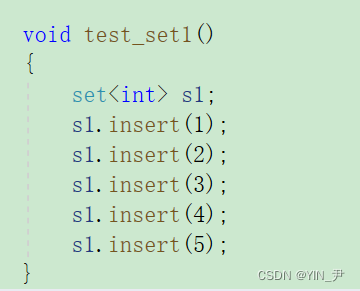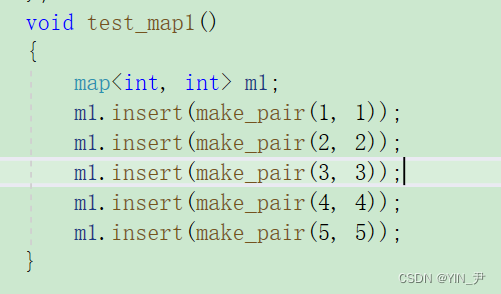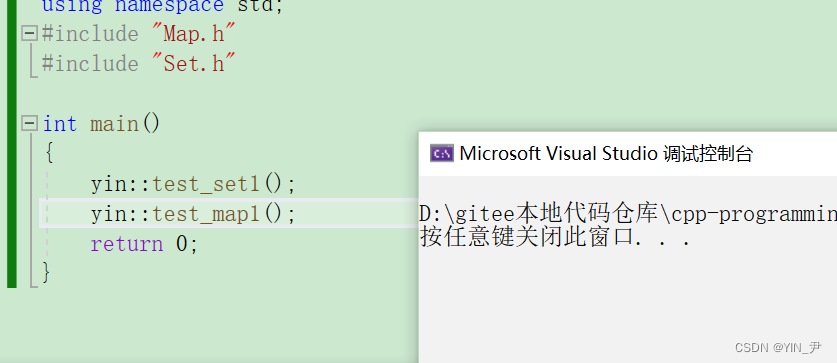## 3.5 发现问题并解决

🆗，我们前面实现的红黑树是K模型的，里面的插入就是按照结点里面的data去比较的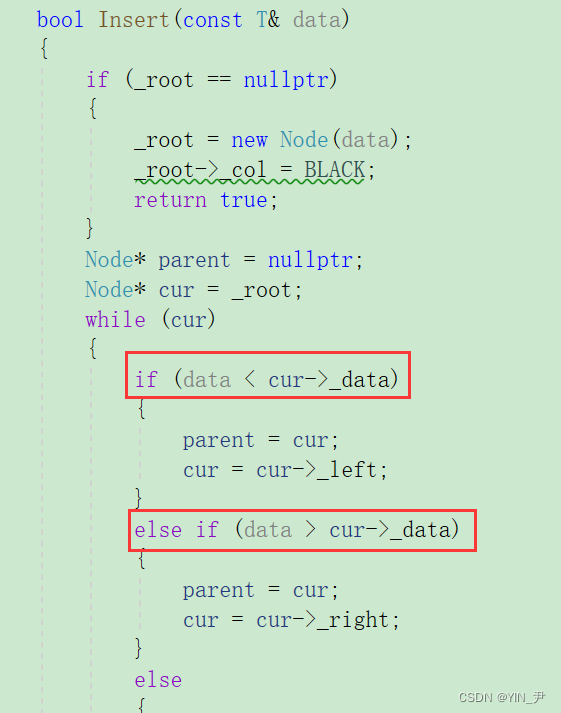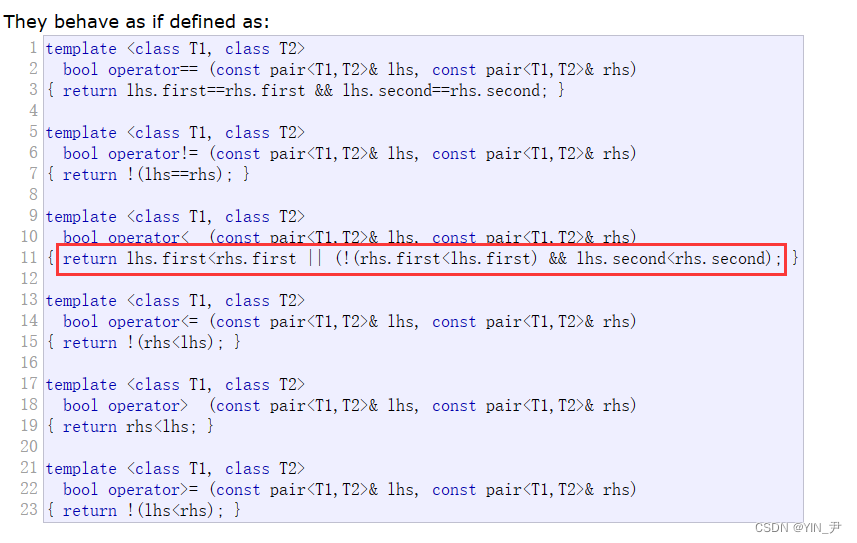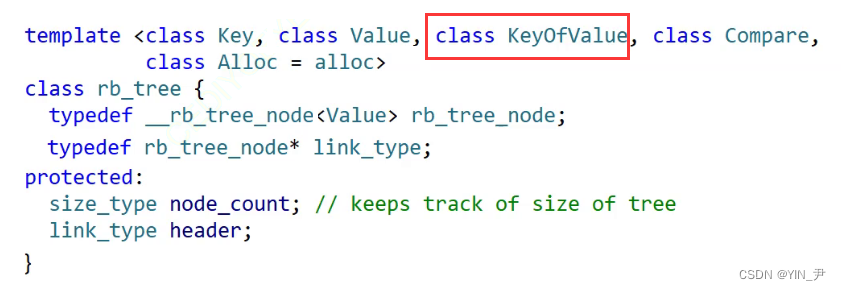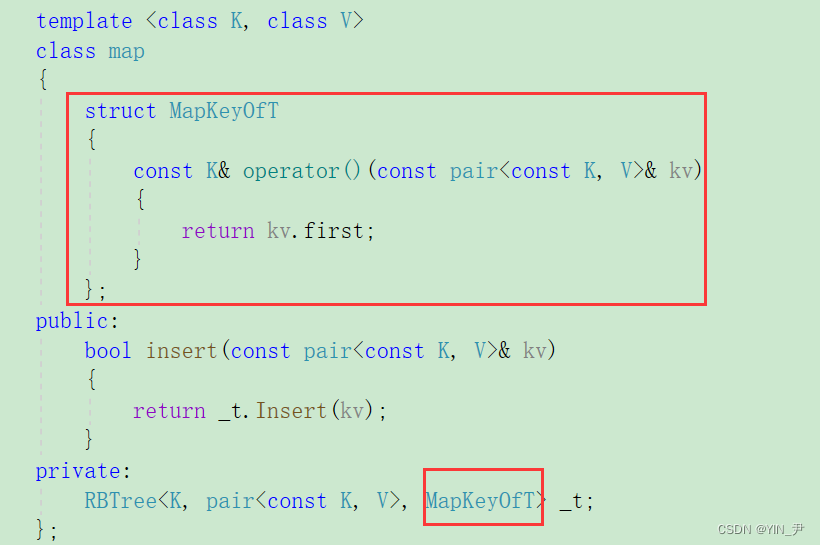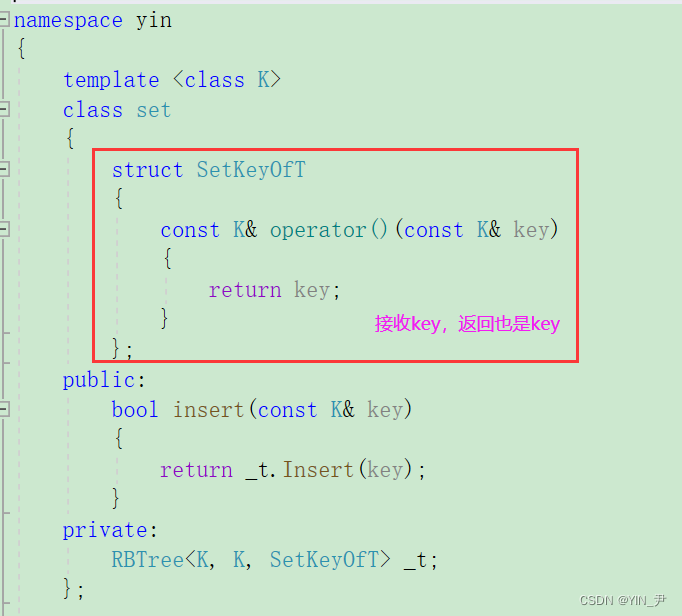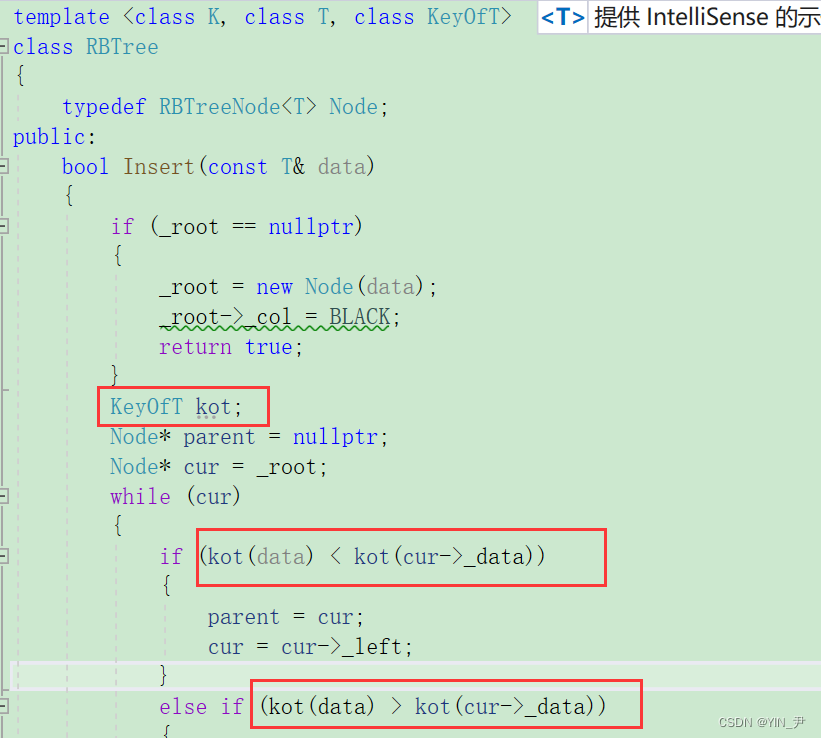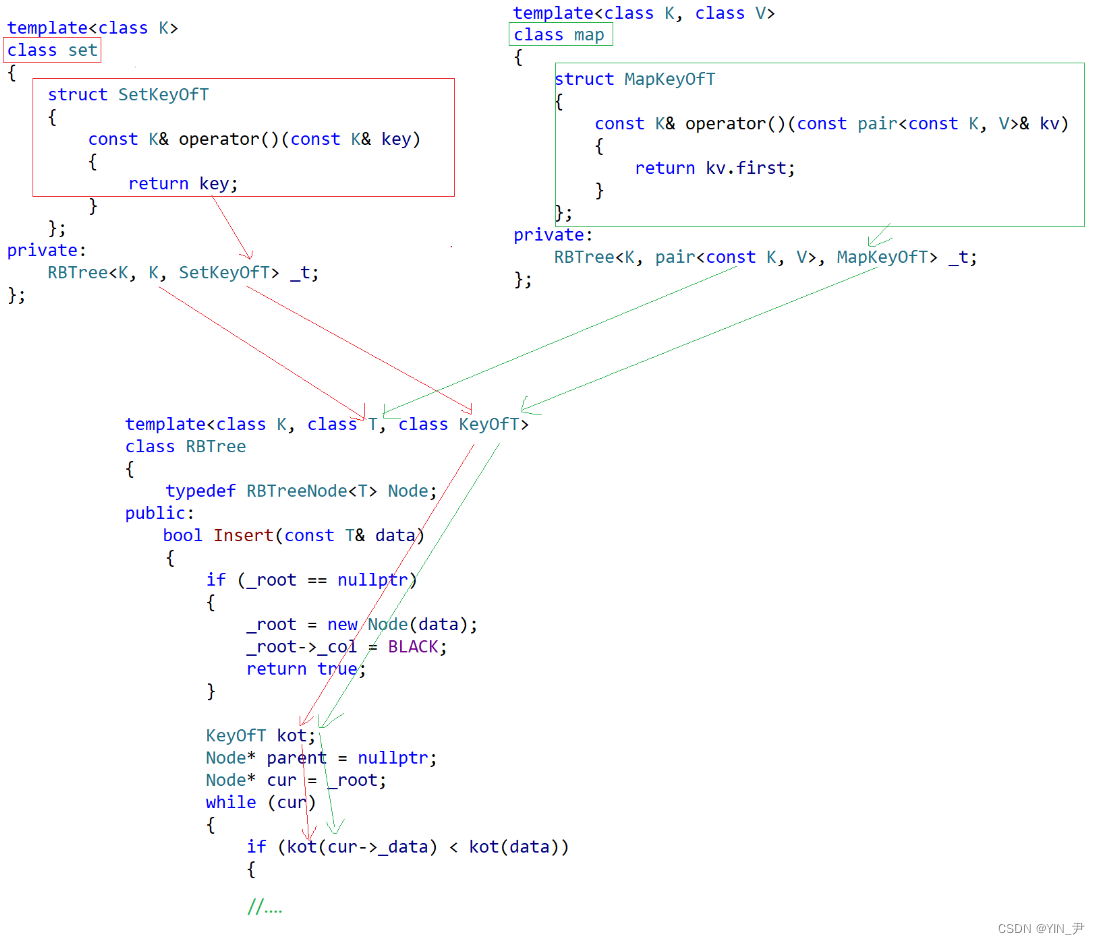🆗，那这个问题我们就解决了

## 3.6 红黑树迭代器实现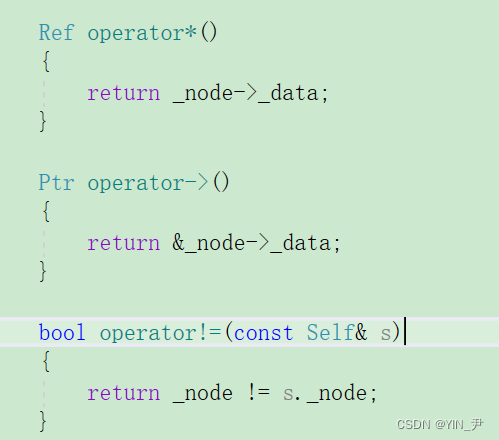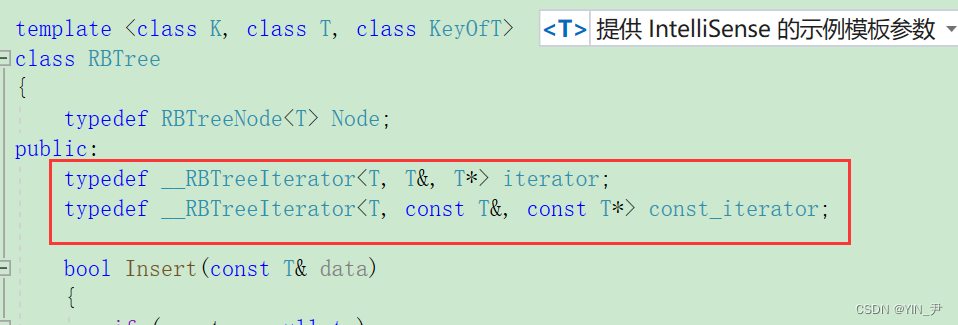🆗，在这里是不是应该返回指向中序遍历第一个结点的迭代器啊。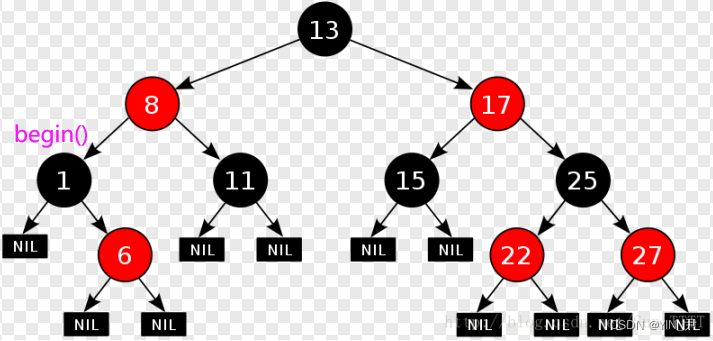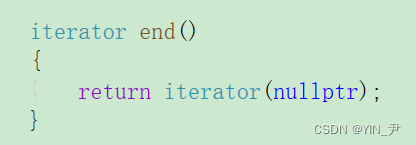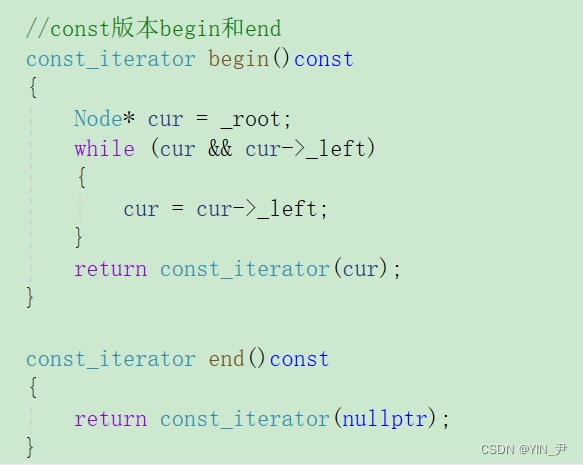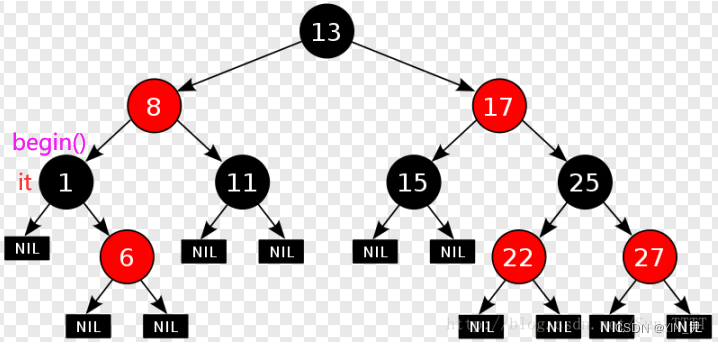🆗，其实不论it当前在那个位置，我们都可以认为它在当前所处的这棵子树的根结点上，那么根结点访问完，中序遍历的话下面访问什么？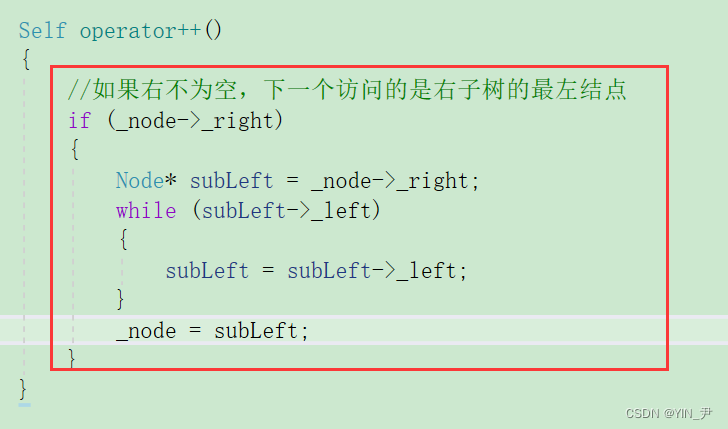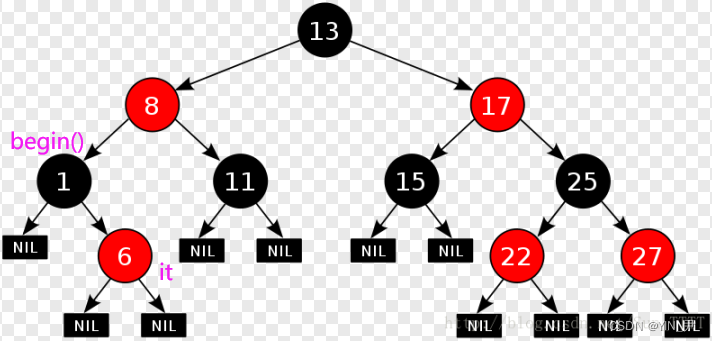🆗，大家想，中序遍历是左、根、右嘛。那66访问完了，6的右为空，那是不是相当于6这棵树访问完了。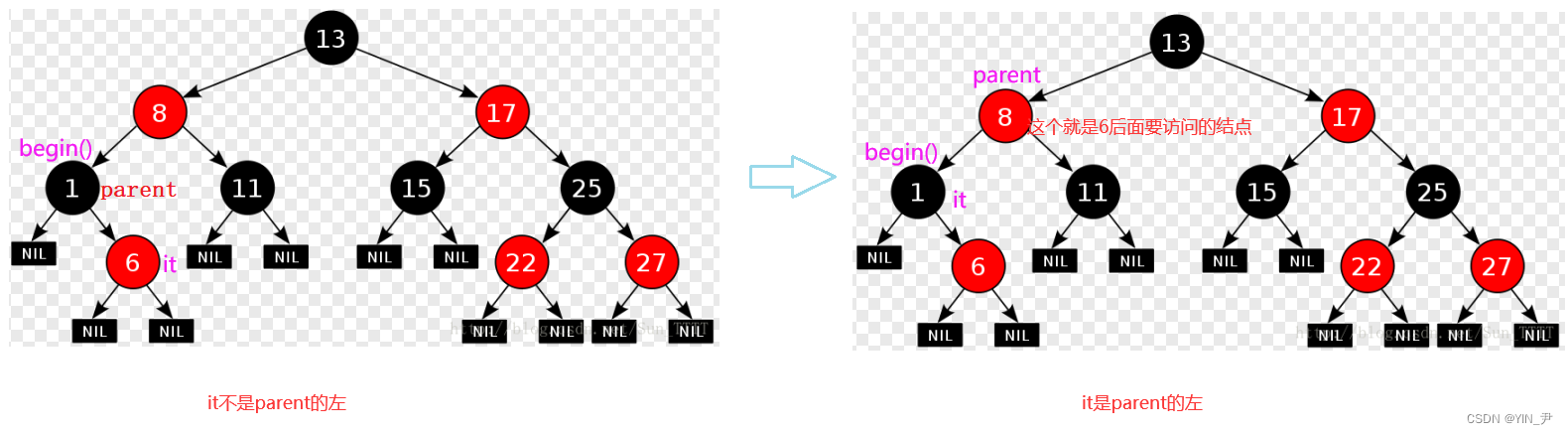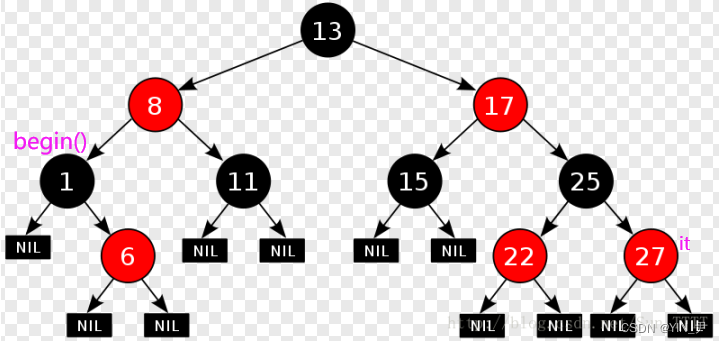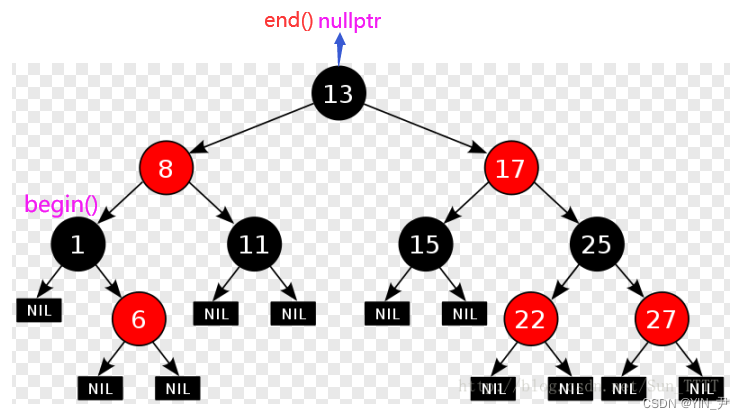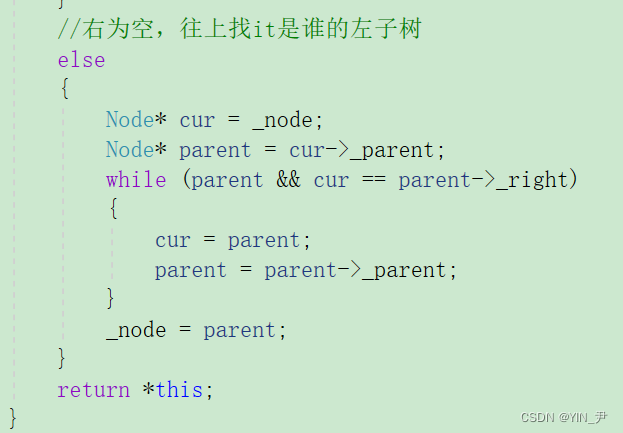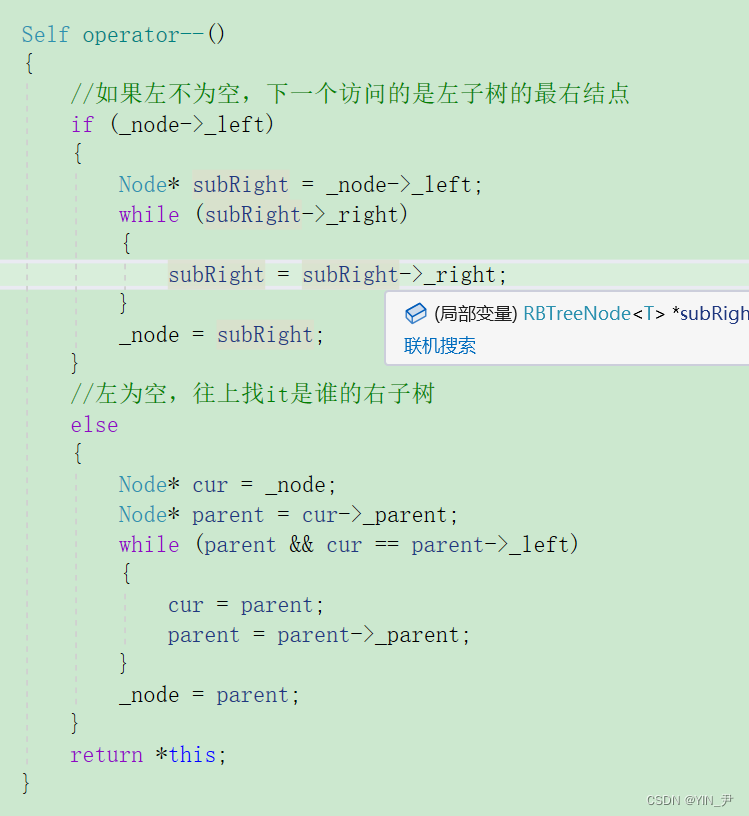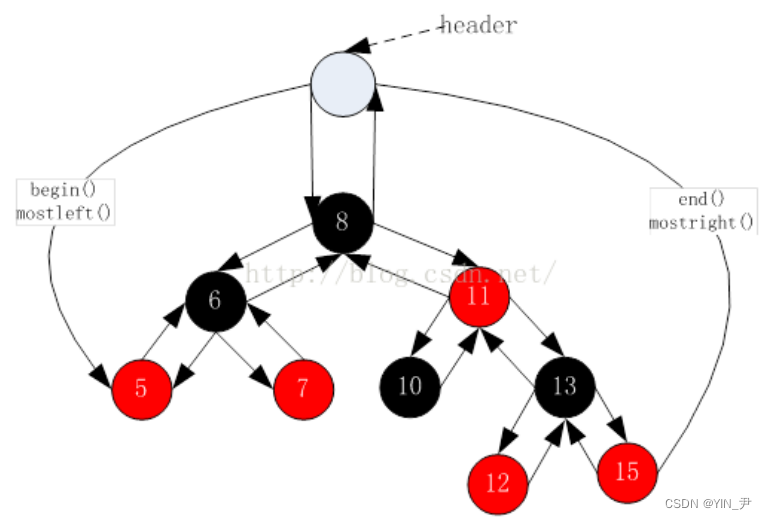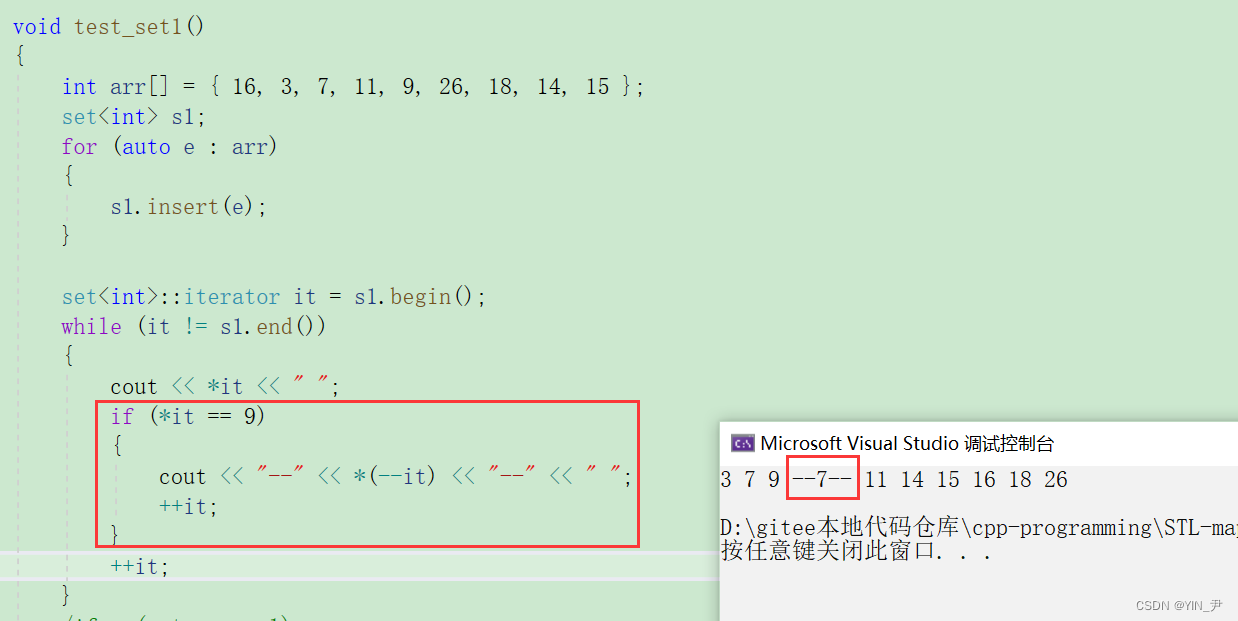## 3.7 封装set和map的迭代器并测试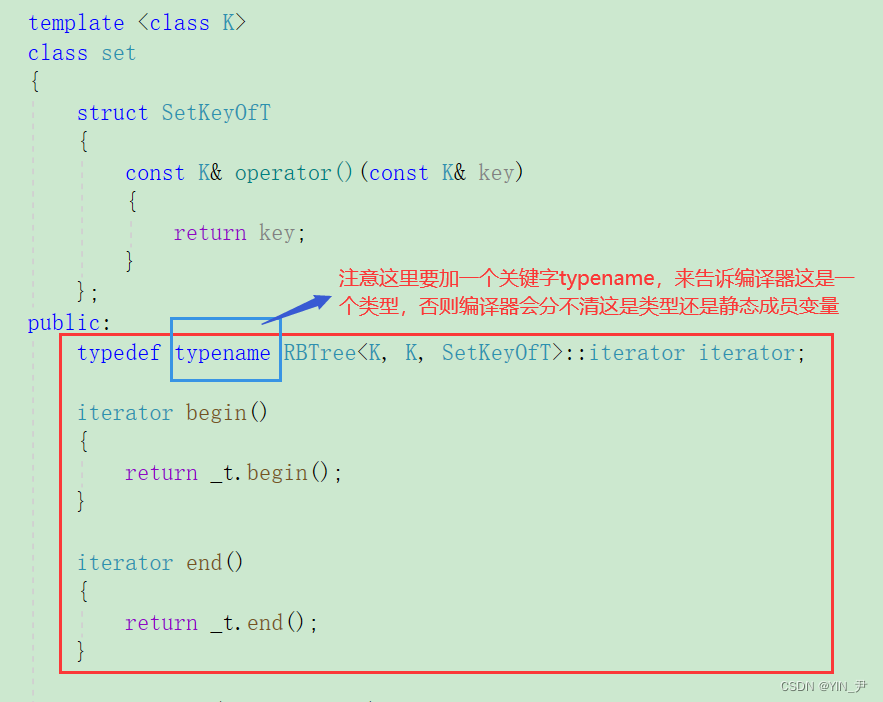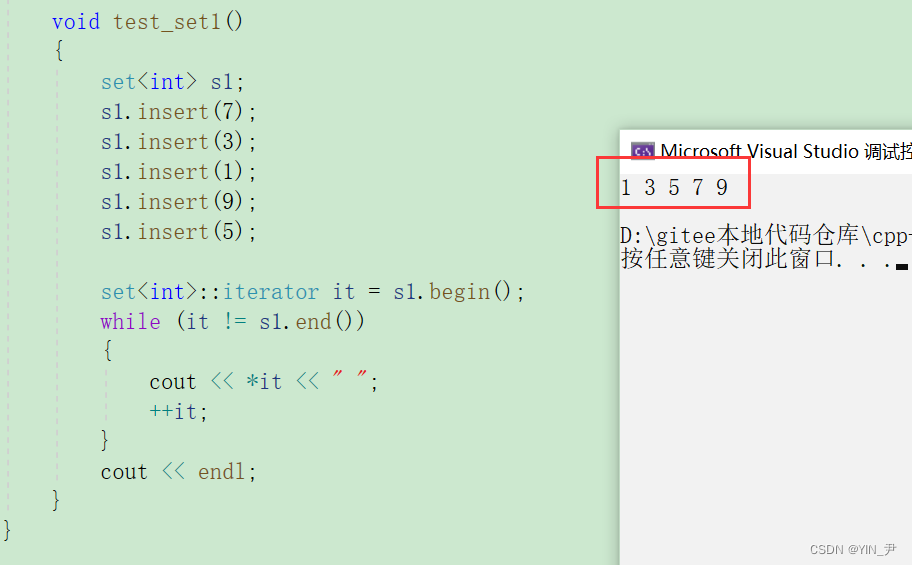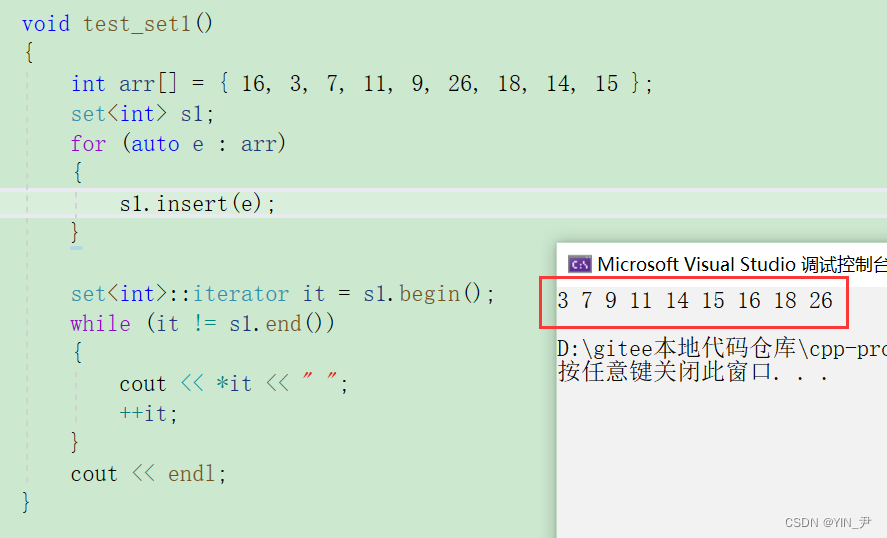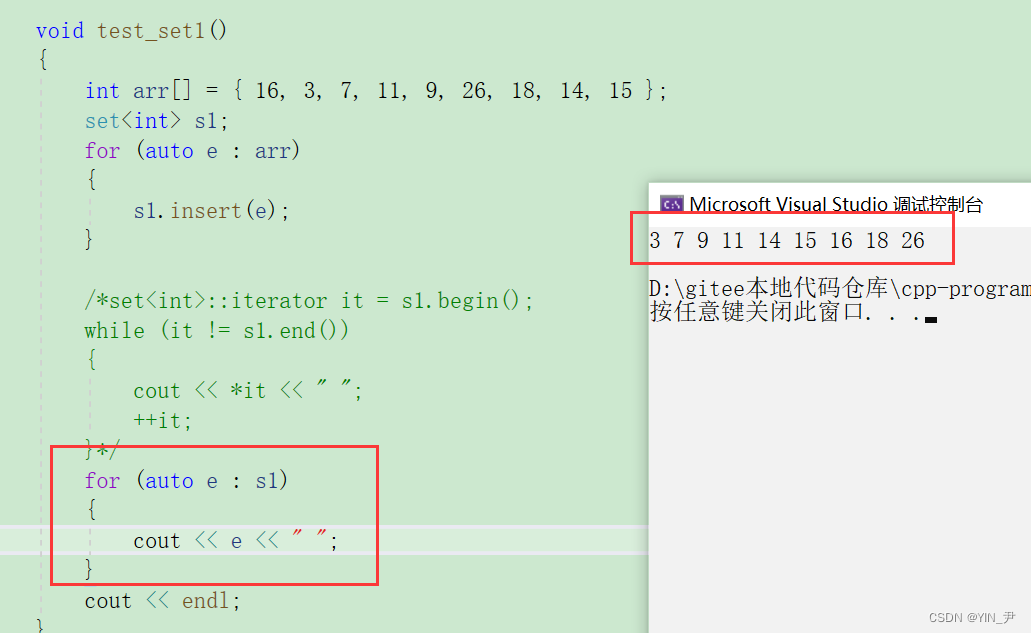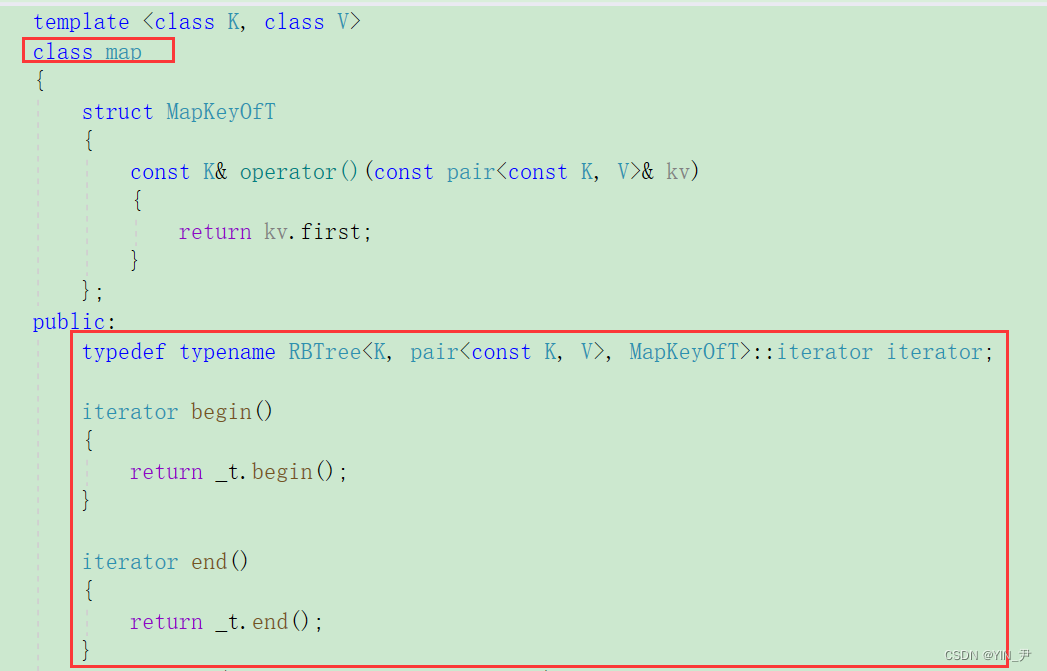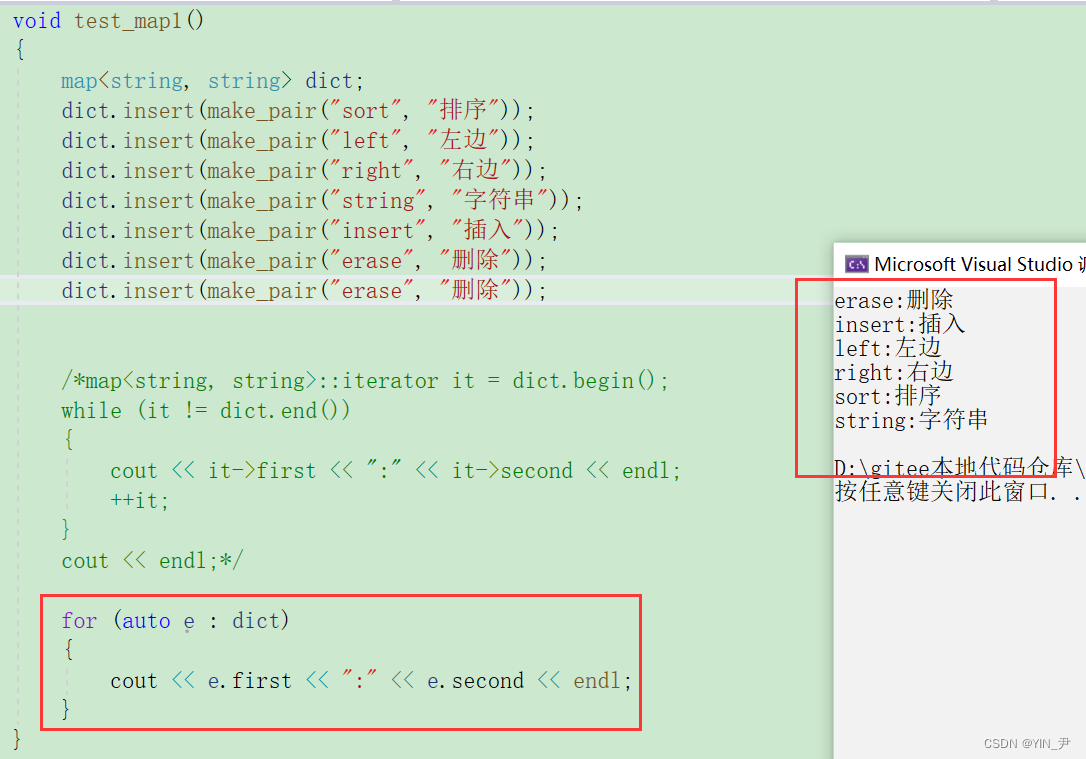## 3.8 map的[]重载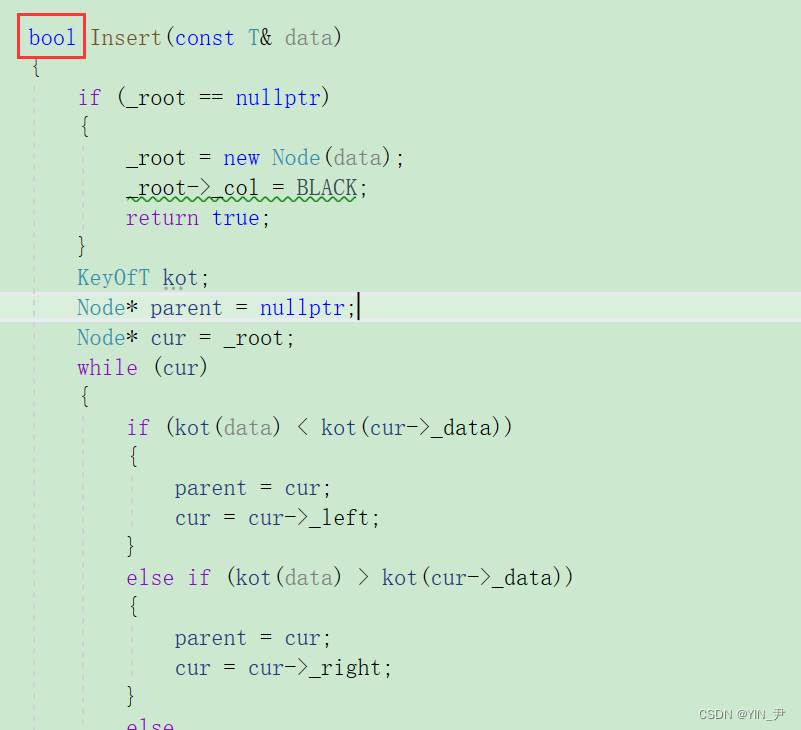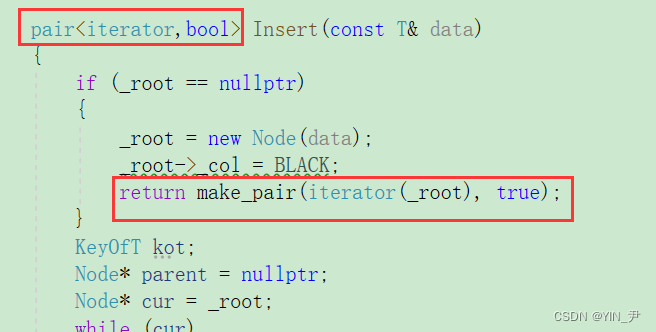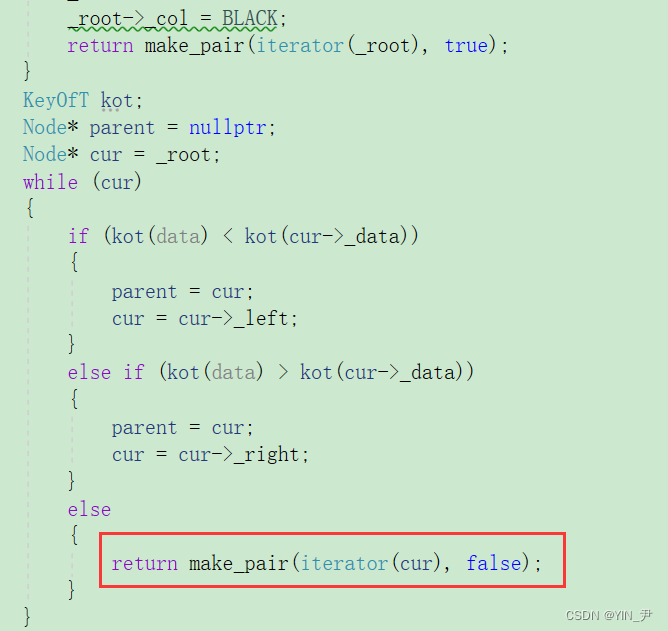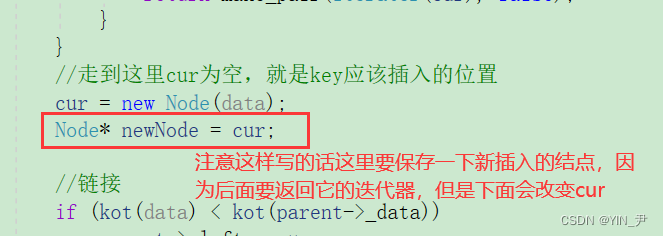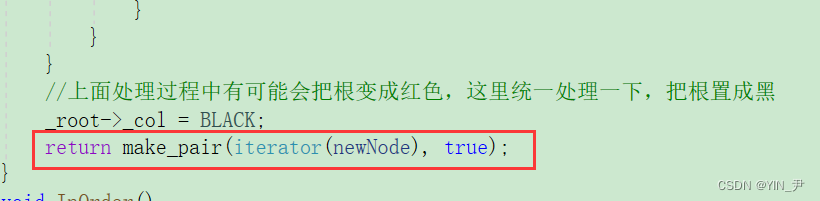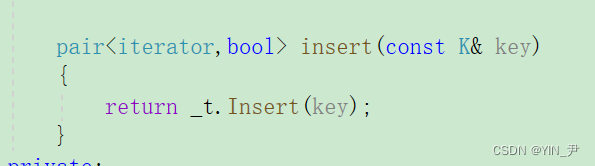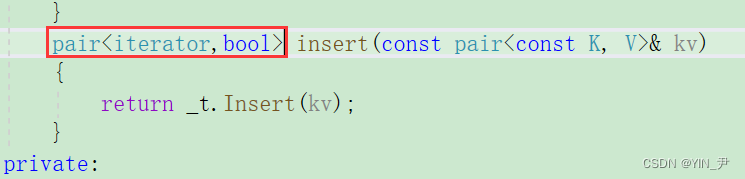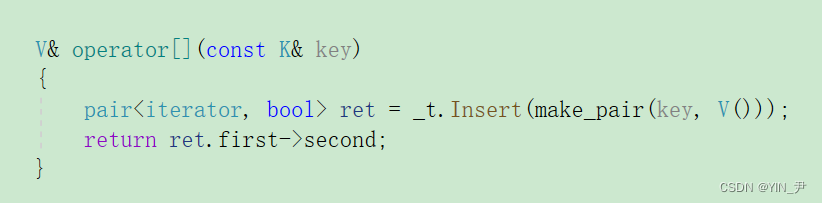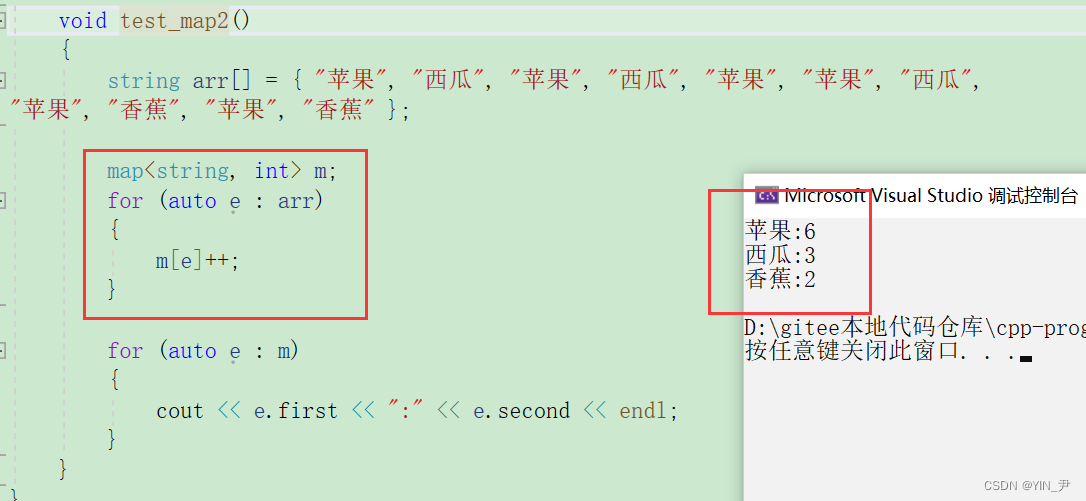## 3.9 元素可以修改的问题解决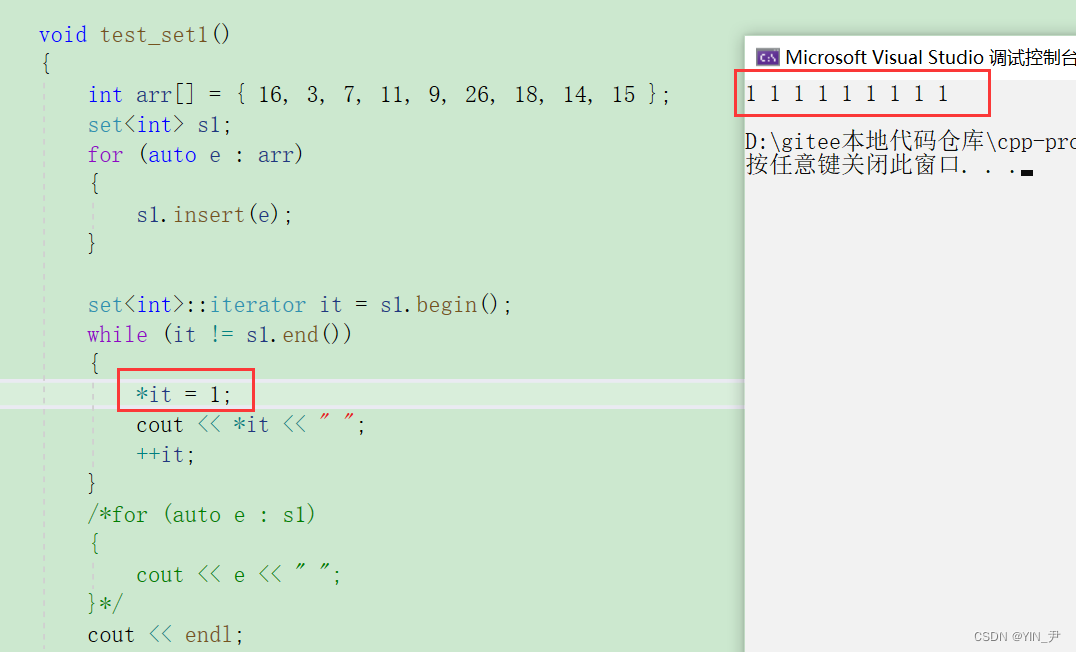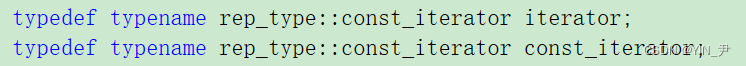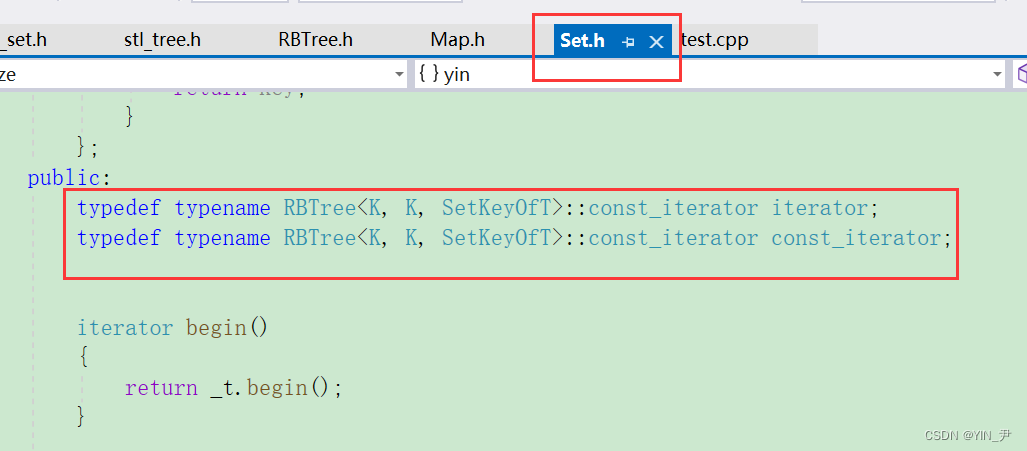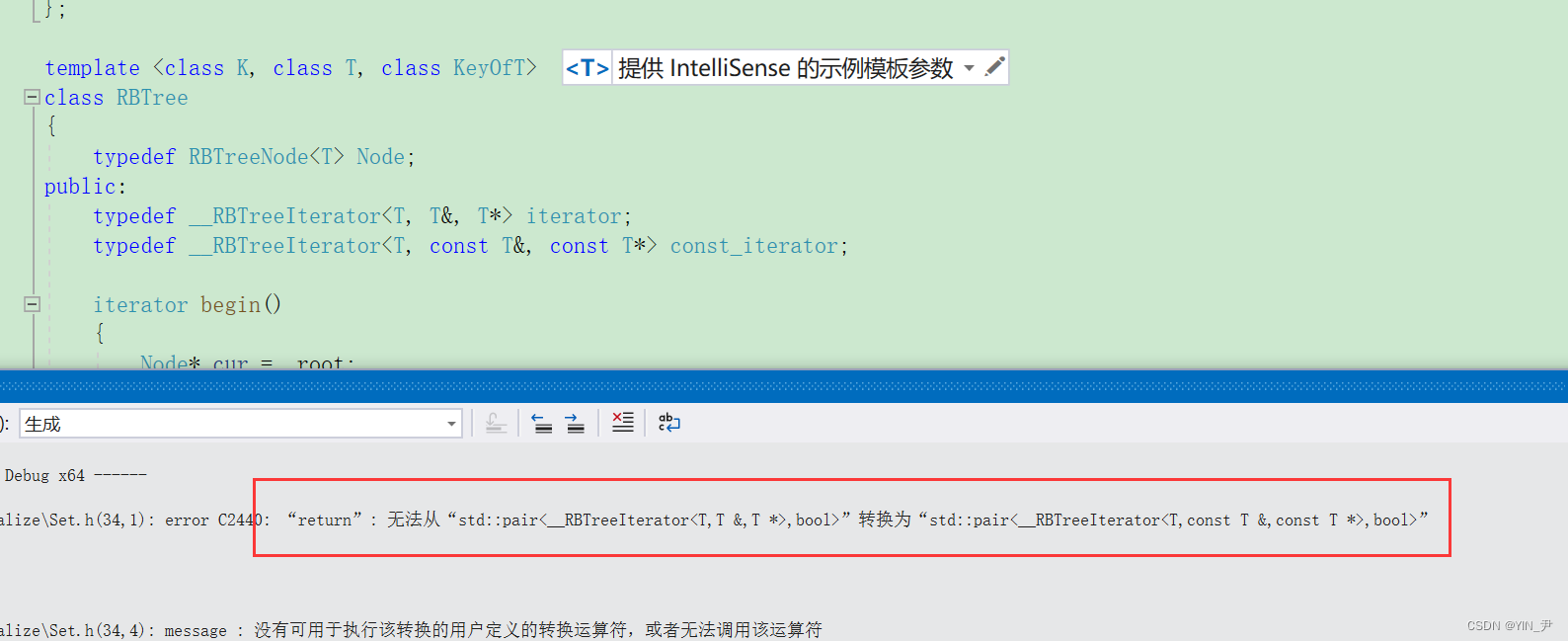🆗，大家看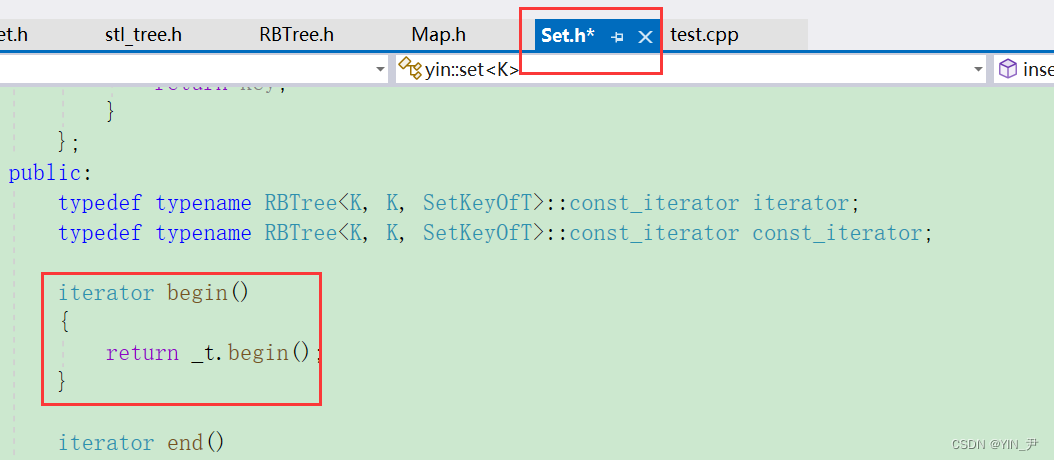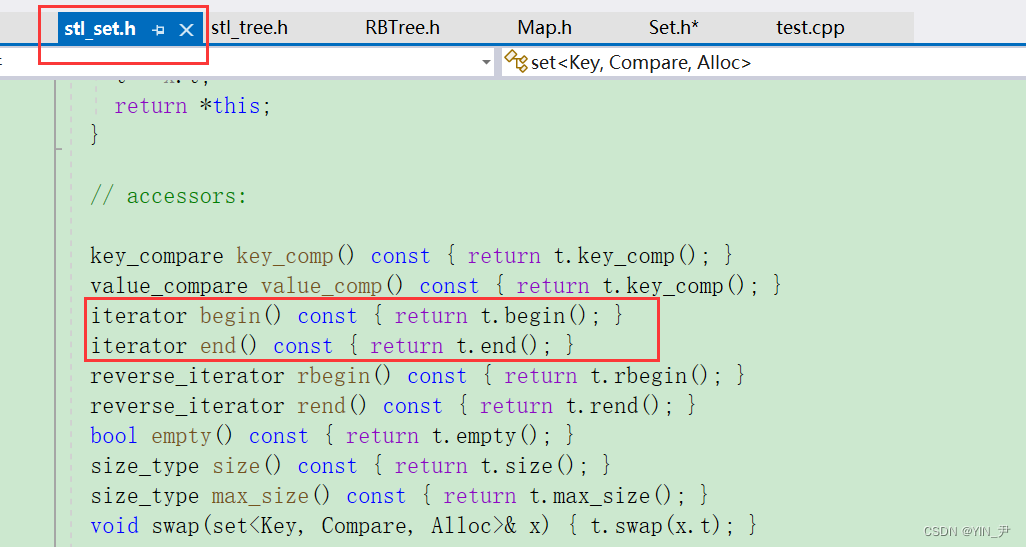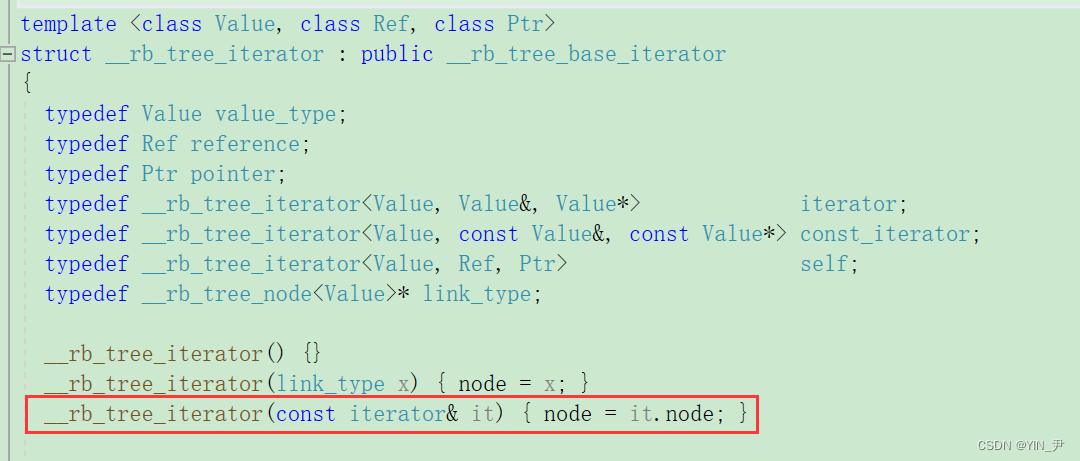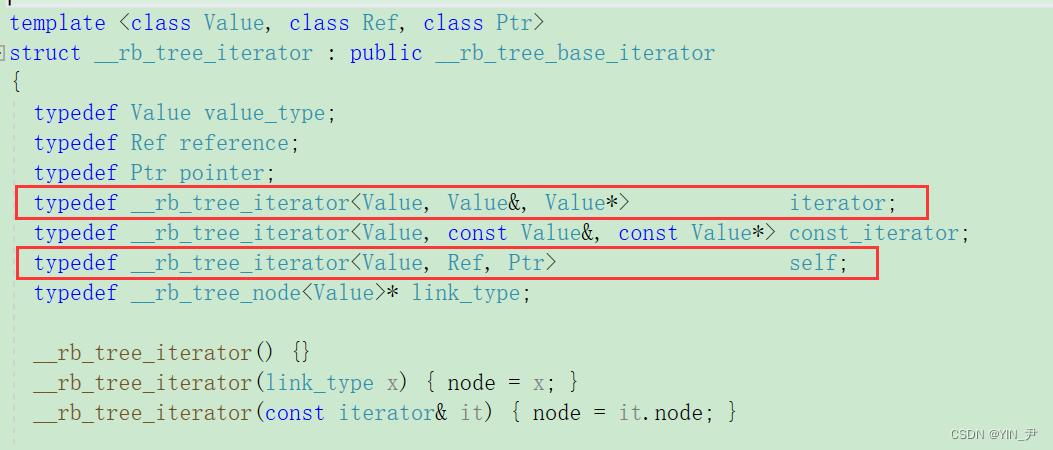Self才是当前类类型的别名，而第三个构造函数的参数是iterator，跟Self是不一样的，如果它用Self的话那就是拷贝构造了。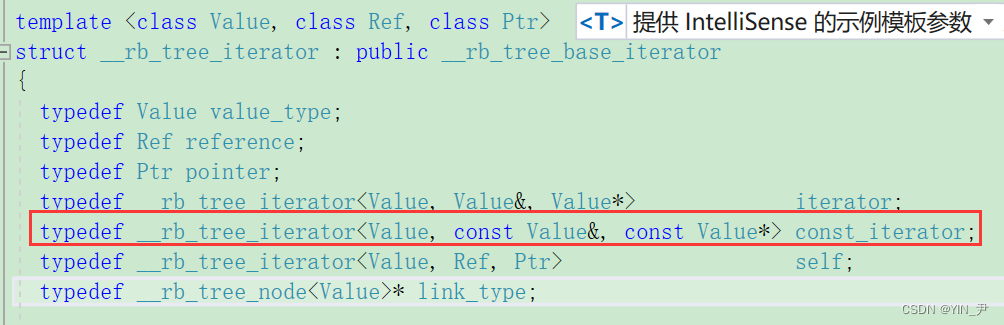`const_iterator`的话，那它就是一个可以用iterator去构造const_iterator的构造函数了。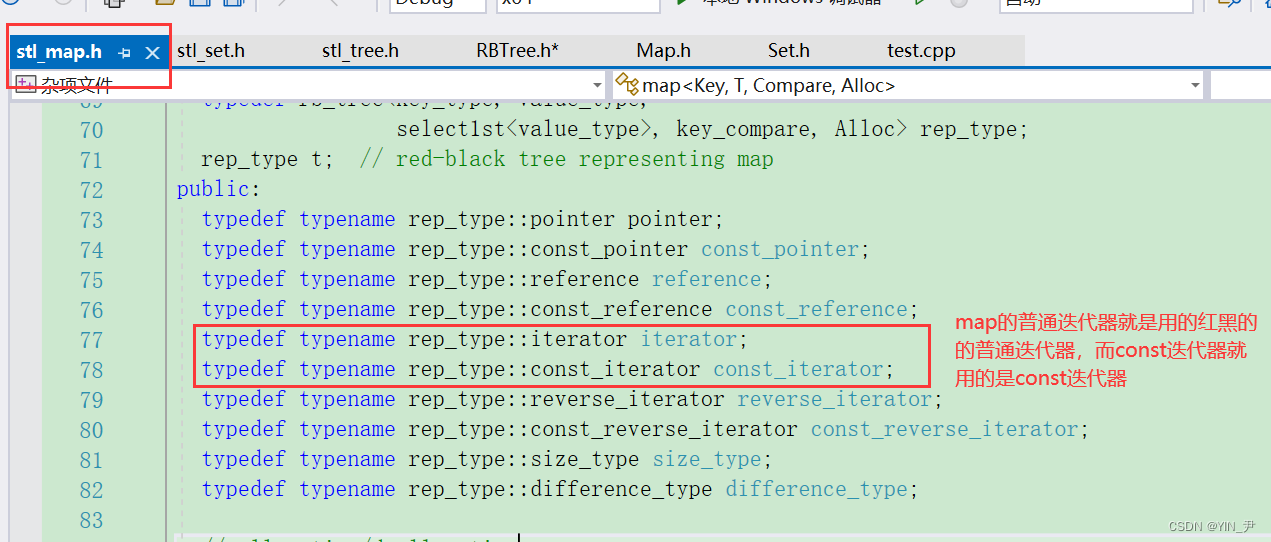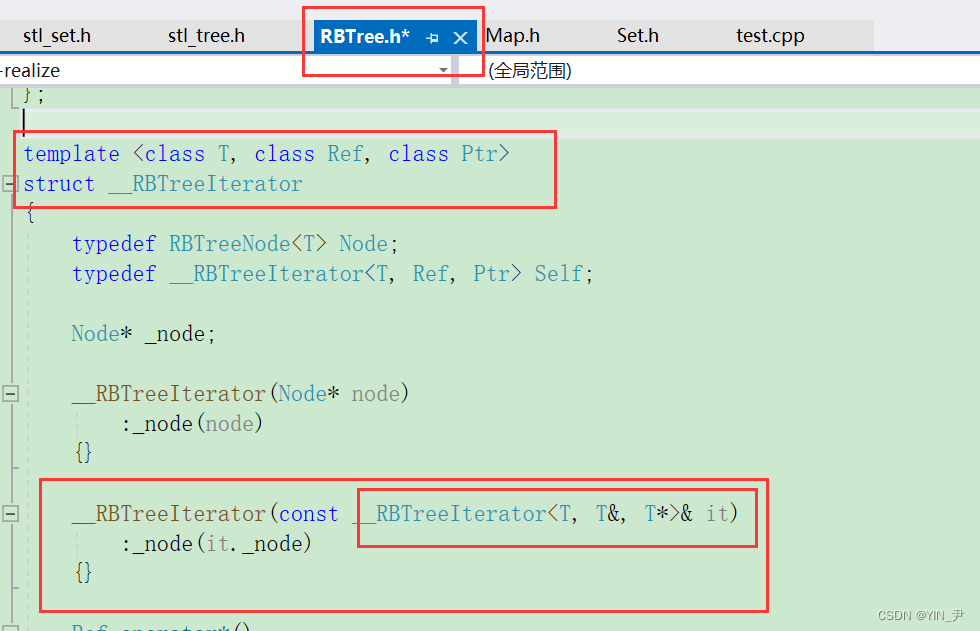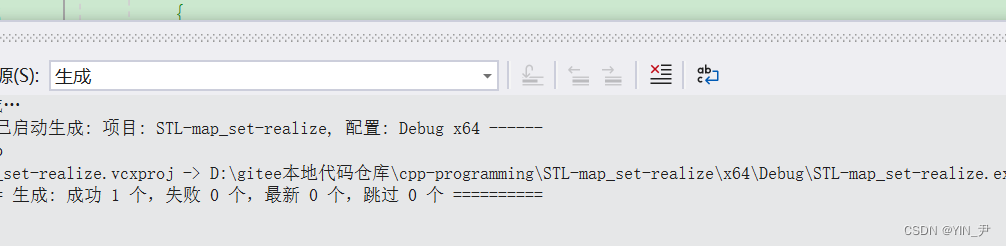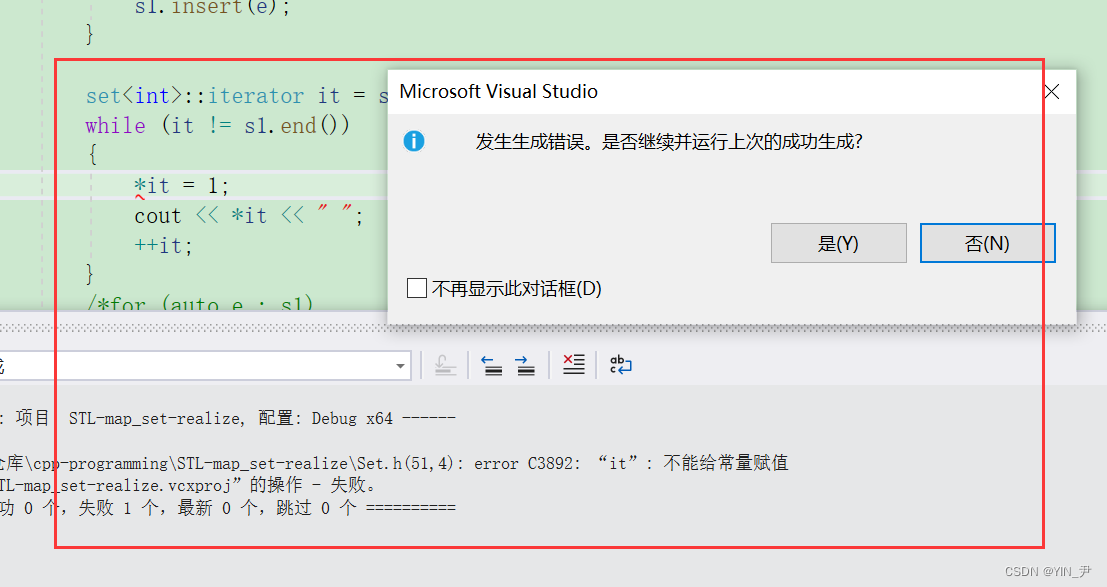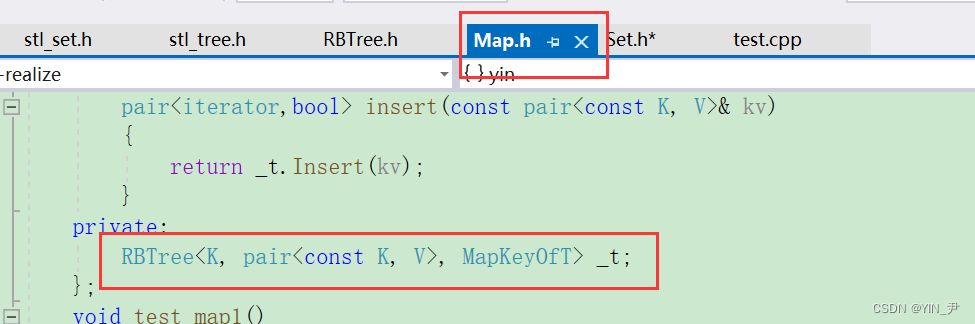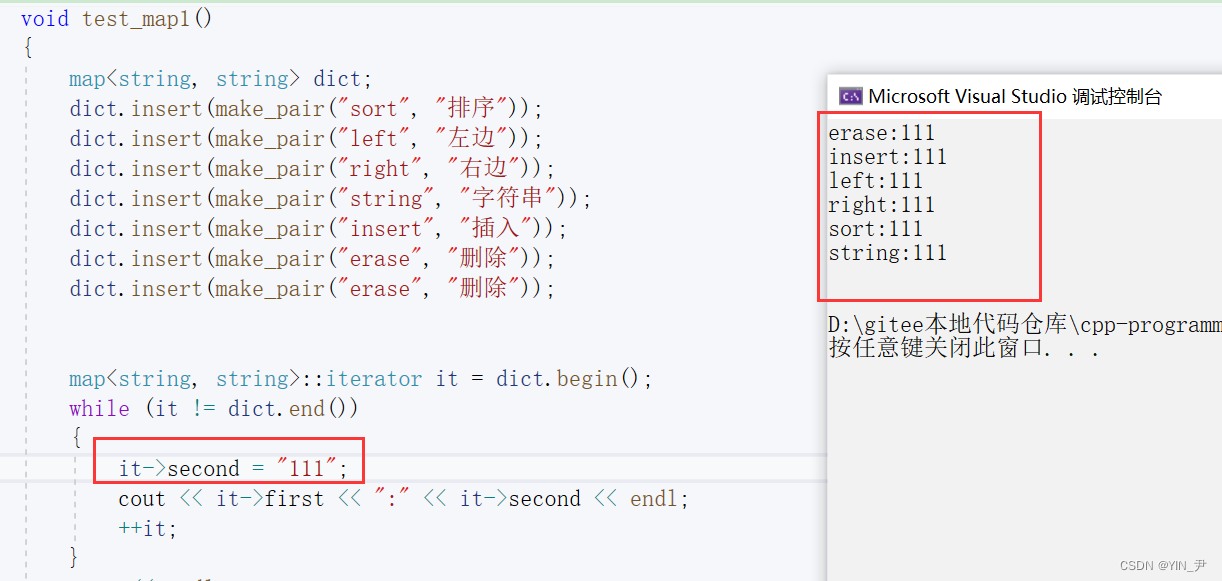second即value是可以修改的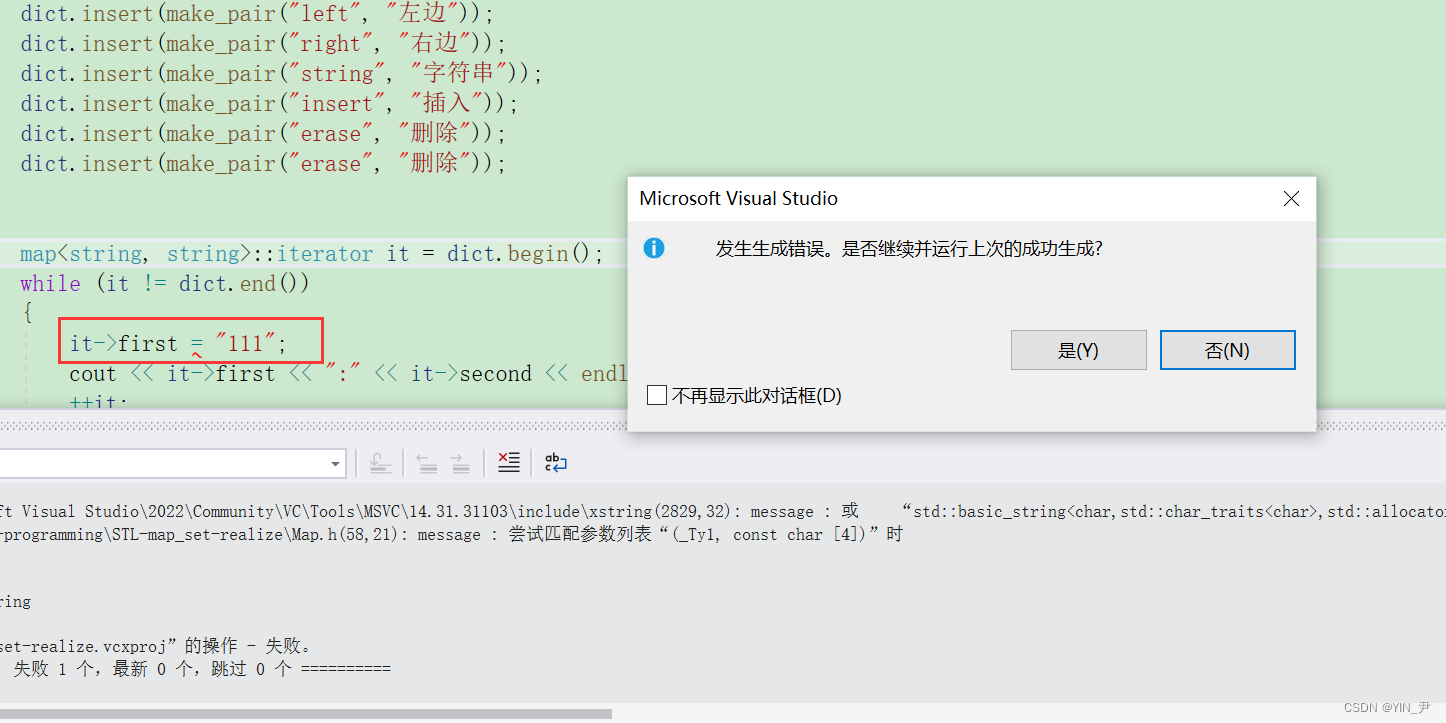🆗，那我们的map和set的模拟实现就差不多讲到这里，其它一些我们这里没实现的东西大家有兴趣的可以自己补充，这里我们就不写了。

# 4. 代码展示

## 4.1 RBTree.h

``````#pragma once

enum Colour
{
RED,
BLACK,
};

template <class T>
struct RBTreeNode
{
RBTreeNode<T>* _parent;
RBTreeNode<T>* _left;
RBTreeNode<T>* _right;
T _data;
Colour _col;

RBTreeNode(const T& data)
:_parent(nullptr)
, _left(nullptr)
, _right(nullptr)
, _data(data)
, _col(RED)
{}

};

template <class T, class Ref, class Ptr>
struct __RBTreeIterator
{
typedef RBTreeNode<T> Node;
typedef __RBTreeIterator<T, Ref, Ptr> Self;

Node* _node;

__RBTreeIterator(Node* node)
:_node(node)
{}

//不论`__RBTreeIterator`被实例化成了普通迭代器还是const迭代器（取决于Ref和Ptr传的是啥）
//，我这里都是用普通迭代器去构造的（因为参数是`<T, T&, T*>`）
__RBTreeIterator(const __RBTreeIterator<T, T&, T*>& it)
:_node(it._node)
{}

Ref operator*()
{
return _node->_data;
}

Ptr operator->()
{
return &_node->_data;
}

bool operator!=(const Self& s)
{
return _node != s._node;
}

Self operator++()
{
//如果右不为空，下一个访问的是右子树的最左结点
if (_node->_right)
{
Node* subLeft = _node->_right;
while (subLeft->_left)
{
subLeft = subLeft->_left;
}
_node = subLeft;
}
//右为空，往上找it是谁的左子树
else
{
Node* cur = _node;
Node* parent = cur->_parent;
while (parent && cur == parent->_right)
{
cur = parent;
parent = parent->_parent;
}
_node = parent;
}
return *this;
}

Self operator--()
{
//如果左不为空，下一个访问的是左子树的最右结点
if (_node->_left)
{
Node* subRight = _node->_left;
while (subRight->_right)
{
subRight = subRight->_right;
}
_node = subRight;
}
//左为空，往上找it是谁的右子树
else
{
Node* cur = _node;
Node* parent = cur->_parent;
while (parent && cur == parent->_left)
{
cur = parent;
parent = parent->_parent;
}
_node = parent;
}
return *this;
}
};

template <class K, class T, class KeyOfT>
class RBTree
{
typedef RBTreeNode<T> Node;
public:
typedef __RBTreeIterator<T, T&, T*> iterator;
typedef __RBTreeIterator<T, const T&, const T*> const_iterator;

iterator begin()
{
Node* cur = _root;
while (cur && cur->_left)
{
cur = cur->_left;
}
return iterator(cur);
}

iterator end()
{
return iterator(nullptr);
}

//const版本begin和end
const_iterator begin()const
{
Node* cur = _root;
while (cur && cur->_left)
{
cur = cur->_left;
}
return const_iterator(cur);
}

const_iterator end()const
{
return const_iterator(nullptr);
}

pair<iterator,bool> Insert(const T& data)
{
if (_root == nullptr)
{
_root = new Node(data);
_root->_col = BLACK;
return make_pair(iterator(_root), true);
}
KeyOfT kot;
Node* parent = nullptr;
Node* cur = _root;
while (cur)
{
if (kot(data) < kot(cur->_data))
{
parent = cur;
cur = cur->_left;
}
else if (kot(data) > kot(cur->_data))
{
parent = cur;
cur = cur->_right;
}
else
{
return make_pair(iterator(cur), false);
}
}
//走到这里cur为空，就是key应该插入的位置
cur = new Node(data);
Node* newNode = cur;

//链接
if (kot(data) < kot(parent->_data))
parent->_left = cur;
if (kot(data) > kot(parent->_data))
parent->_right = cur;

//链接父亲指针
cur->_parent = parent;

//如果插入之后它的parent是红的，就需要进行调整
while (parent && parent->_col == RED)
{
Node* grandfather = parent->_parent;
//如果父亲是祖父的左孩子，那右孩子就是叔叔
if (parent == grandfather->_left)
{
Node* uncle = grandfather->_right;
//这里处理的情况是叔叔存在且为红，变色+向上继续处理
if (uncle && uncle->_col == RED)
{
//将p,u改为黑，g改为红
parent->_col = BLACK;
uncle->_col = BLACK;
grandfather->_col = RED;

//更新cur为grandfather，判断它的父亲是什么情况：
//1.如果不存在或者为黑，就需要继续处理了，也不会进行循环了
//2.如果它的父亲存在且为红，重新循环进行调整
cur = grandfather;
parent = cur->_parent;
}
else//叔叔不存在/叔叔存在且为黑的情况
{
//     g
//   p   u
// c
if (cur == parent->_left)//左左——右单旋+变色
{
RotateR(grandfather);
parent->_col = BLACK;
grandfather->_col = RED;
}
else//左右——左右双旋+变色
{
//     g
//   p   u
//     c
RotateL(parent);
RotateR(grandfather);
cur->_col = BLACK;
grandfather->_col = RED;
}
//这里调整完就可以break了，因为颜色都变的合适了，而相关的链接关系旋转会帮我们处理
break;
}
}
//如果父亲是祖父的右孩子，那左孩子就是叔叔
else //parent = grandfather->_right
{
Node* uncle = grandfather->_left;
//这里处理的情况是叔叔存在且为红
if (uncle && uncle->_col == RED)
{
//将p,u改为黑，g改为红
parent->_col = BLACK;
uncle->_col = BLACK;
grandfather->_col = RED;

//更新cur为grandfather，判断它的父亲是什么情况：
//1.如果不存在或者为黑，就需要继续处理了，也不会进行循环了
//2.如果它的父亲存在且为红，重新循环进行调整
cur = grandfather;
parent = cur->_parent;
}
else
{
if (cur == parent->_right)//右右——左单旋+变色
{
//    g
//  u   p
//        c
RotateL(grandfather);
parent->_col = BLACK;
grandfather->_col = RED;
}
else//右左——右左双旋+变色
{
//    g
//  u   p
//    c
RotateR(parent);
RotateL(grandfather);
cur->_col = BLACK;
grandfather->_col = RED;
}
break;
}
}
}
//上面处理过程中有可能会把根变成红色，这里统一处理一下，把根置成黑
_root->_col = BLACK;
return make_pair(iterator(newNode), true);
}
void InOrder()
{
_InOrder(_root);
cout << endl;
}
bool IsBlance()
{
if (_root && _root->_col == RED)
{
cout << "根结点是红色" << endl;
return false;
}

//先求出一条路径黑色结点数量
int mark = 0;
Node* cur = _root;
while (cur)
{
if (cur->_col == BLACK)
++mark;
cur = cur->_left;
}

//检查是否出现连续红色结点及所有路径黑色结点数量是否相等
return _Check(_root, 0, mark);
}
int TreeHeight()
{
return _TreeHeight(_root);
}
Node* find(const T& data)
{
KeyOfT kot;
Node* cur = _root;
while (cur)
{
if (kot(data) < kot(cur->_data))
{
cur = cur->_left;
}
else if (kot(data) > kot(cur->_data))
{
cur = cur->_right;
}
else
{
return cur;
}
}
return nullptr;
}
~RBTree()
{
_Destroy(_root);
_root = nullptr;
}
private:
void _Destroy(Node* root)
{
if (root == nullptr)
return;

_Destroy(root->_left);
_Destroy(root->_right);
delete root;
}
int _TreeHeight(Node* root)
{
if (root == nullptr)
return 0;
int RightH = _TreeHeight(root->_left);
int leftH = _TreeHeight(root->_right);
return RightH > leftH ? RightH + 1 : leftH + 1;
}
bool _Check(Node* root, int blackNum, int mark)
{
if (root == nullptr)
{
//走到空就是一条路径走完了，比较一下是否相等
if (blackNum != mark)
{
cout << "存在黑色结点数量不相等" << endl;
return false;
}
return true;
}

if (root->_col == BLACK)
++blackNum;

if (root->_col == RED && root->_parent->_col == RED)
{
cout << "出现连续红色结点" << endl;
return false;
}

return _Check(root->_left, blackNum, mark)
&& _Check(root->_left, blackNum, mark);
}
void _InOrder(Node* root)
{
if (root == nullptr)
{
return;
}
_InOrder(root->_left);
cout << root->_data << " ";
_InOrder(root->_right);
}
//左单旋
void RotateL(Node* parent)
{
Node* subR = parent->_right;
Node* subRL = subR->_left;

//旋转并更新_parent指针
parent->_right = subRL;
if (subRL)
subRL->_parent = parent;

//先保存一下parent->_parent，因为下面会改它
Node* pparent = parent->_parent;

//旋转并更新_parent指针
subR->_left = parent;
parent->_parent = subR;

//若pparent为空则证明旋转的是一整棵树，因为根结点的_parent为空
if (pparent == nullptr)
{
//subR是新的根
_root = subR;
_root->_parent = nullptr;
}
//若pparent不为空，则证明旋转的是子树，parent上面还有结点
else
{
//让pparent指向子树旋转之后新的根
if (pparent->_left == parent)
{
pparent->_left = subR;
}
else
{
pparent->_right = subR;
}
//同时也让新的根指向pparent
subR->_parent = pparent;
}
}

//右单旋
void RotateR(Node* parent)
{
Node* subL = parent->_left;
Node* subLR = subL->_right;

//旋转并更新_parent指针
parent->_left = subLR;
if (subLR)
subLR->_parent = parent;

//先保存一下parent->_parent，因为下面会改它
Node* pparent = parent->_parent;

//旋转并更新_parent指针
subL->_right = parent;
parent->_parent = subL;

//若parent等于_root则证明旋转的是一整棵树（这也是一种判断方法）
if (parent == _root)
{
_root = subL;
_root->_parent = nullptr;
}
else
{
//让pparent指向子树旋转之后新的根
if (parent == pparent->_left)
{
pparent->_left = subL;
}
else
{
pparent->_right = subL;
}
//同时也让新的根指向pparent
subL->_parent = pparent;
}
}

private:
Node* _root = nullptr;
};
``````

## 4.2 Map.h

``````#pragma once

#include "RBTree.h"

namespace yin
{
template <class K, class V>
class map
{
struct MapKeyOfT
{
const K& operator()(const pair<const K, V>& kv)
{
return kv.first;
}
};
public:
typedef typename RBTree<K, pair<const K, V>, MapKeyOfT>::iterator iterator;

iterator begin()
{
return _t.begin();
}

iterator end()
{
return _t.end();
}

V& operator[](const K& key)
{
pair<iterator, bool> ret = _t.Insert(make_pair(key, V()));
return ret.first->second;
}

pair<iterator,bool> insert(const pair<const K, V>& kv)
{
return _t.Insert(kv);
}
private:
RBTree<K, pair<const K, V>, MapKeyOfT> _t;
};
void test_map1()
{
map<string, string> dict;
dict.insert(make_pair("sort", "排序"));
dict.insert(make_pair("left", "左边"));
dict.insert(make_pair("right", "右边"));
dict.insert(make_pair("string", "字符串"));
dict.insert(make_pair("insert", "插入"));
dict.insert(make_pair("erase", "删除"));
dict.insert(make_pair("erase", "删除"));

map<string, string>::iterator it = dict.begin();
while (it != dict.end())
{
cout << it->first << ":" << it->second << endl;
++it;
}
cout << endl;

/*for (auto e : dict)
{
cout << e.first << ":" << e.second << endl;
}*/
}
void test_map2()
{
string arr[] = { "苹果", "西瓜", "苹果", "西瓜", "苹果", "苹果", "西瓜",
"苹果", "香蕉", "苹果", "香蕉" };

map<string, int> m;
for (auto e : arr)
{
m[e]++;
}

for (auto e : m)
{
cout << e.first << ":" << e.second << endl;
}
}
}
``````

## 4.3 Set.h

``````#pragma once

#include "RBTree.h"

namespace yin
{
template <class K>
class set
{
struct SetKeyOfT
{
const K& operator()(const K& key)
{
return key;
}
};
public:
typedef typename RBTree<K, K, SetKeyOfT>::const_iterator iterator;
typedef typename RBTree<K, K, SetKeyOfT>::const_iterator const_iterator;

iterator begin()
{
return _t.begin();
}

iterator end()
{
return _t.end();
}

pair<iterator,bool> insert(const K& key)
{
return _t.Insert(key);
}
private:
RBTree<K, K, SetKeyOfT> _t;
};

void test_set1()
{
int arr[] = { 16, 3, 7, 11, 9, 26, 18, 14, 15 };
set<int> s1;
for (auto e : arr)
{
s1.insert(e);
}

set<int>::iterator it = s1.begin();
while (it != s1.end())
{
cout << *it << " ";
++it;
}
/*for (auto e : s1)
{
cout << e << " ";
}*/
cout << endl;
}
}
``````

## 4.4 Test.cpp

``````#define _CRT_SECURE_NO_WARNINGS
#include <iostream>
using namespace std;
#include "Map.h"
#include "Set.h"
#include <set>

int main()
{
//yin::test_set1();
yin::test_map1();
return 0;
}
``````### svg图片如何渲染到页面，以及svg文件的上传

svg图片渲染到页面的几种方式 背景&#x1f7e1;require.context获取目录下的所有文件&#x1f7e1;方式1: 直接在html中渲染&#x1f7e1;方式: 发起ajax请求&#xff0c;获取SVG文件 背景 需要实现从本地目录下去获取所有的svg图标进行预览&#xff0c;将选中的图片显示在另…

### Dockerfile制作镜像与搭建LAMP环境

1、编写Dockerfile制作Web应用系统nginx镜像&#xff0c;生成镜像nginx:v1.1&#xff0c;并推送其到私有仓库。 具体要求如下&#xff1a; &#xff08;1&#xff09;基于centos基础镜像&#xff1b; &#xff08;2&#xff09;指定作者信息&#xff1b; &#xff08;3&#x…

### AveMaria 传播手段的变化

AveMaria 是一种最早在 2018 年 12 月出现的窃密木马&#xff0c;攻击者越来越喜欢使用其进行攻击&#xff0c;运营方也一直在持续更新和升级。在过去六个月中&#xff0c;研究人员观察到 AveMaria 的传播手段发生了许多变化。 2022 年 12 月攻击行动 研究人员发现了名为 .Vh…